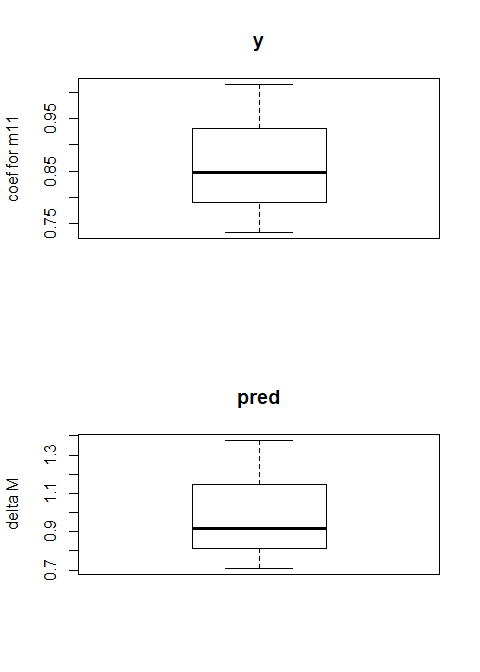# Examples for Big Data Mediation Analysis

## Package installation

R package mmabig is used for general multiple mediation/confounding analysis with high dimensional data sets (Li et al. 2018). Mediation/confounding effects refer to the effects from third variables that intervene the relationship between an exposure and an outcome. In the following we generally call the mediation/confounding effect as the third-variable effect (TVE). TVEs include the total effect between the exposure and the outcome, direct effect from the exposure to outcome after adjusting for other variables, and indirect effect of a third variable (the effect from the exposure variable to the third variable and to the outcome), which are defined and the statistical inferences described in Li et al. (2018). In mmabig, a generalized linear model with LASSO or elastic net regularization is used to fit the final model. If readers are interested in using a generalized linear model or multiple Additive Regression Trees (MART) to fit the model, please refer to the R package mma (Yu and Li 2017).

To use the R package mmabig, we first install the package in R (install.packages("mmabig")) and load it.

## Data Organization and Identify Potential Moderators/Confounders

The function data.org.big is used to do a preliminary data analysis to identify potential Mediators/Confounders (MCs) and covariates. It returns an object of “med_iden” class that organizes the data into a format to be used directly for the mediation analysis functions.

### One binary/continuous predictor and one binary/continuous outcome

The following code generates a simulated data set with 20 potential MCs, of which five (m16-m20) are multicategorical variables. The real mediator/confounders are “m11”, “m12”, and “m16”, which are highly related with both the predictor “pred” and the outcome “y”.

# a binary predictor
set.seed(1)
n=100
pred=rbinom(n,1,0.5)
m1=matrix(rnorm(n*10),n,10)
m2<-matrix(rnorm(n*10,mean=pred,sd=1),n,10)
m3.1=m2[,6:10]
m3=m3.1
m2=m2[,1:5]
m3[m3.1<=0.1]=0
m3[0.1<m3.1 & m3.1<=1]=1
m3[m3.1>1]=2
m3<-apply(m3,2,as.factor)
m<-data.frame(m1,m2,m3)
colnames(m)<-c(paste("m0",1:9,sep=""),paste("m",10:20,sep=""))

lu<--0.5363+0.701*pred+0.801*m[,1]+0.518*m[,2]+1.402*m[,11]+0.773*m[,12]+
ifelse(m[,16]=="2",2.15,0)+ifelse(m[,16]=="1",0.201,0)

# a continuous y
y<-rnorm(n,lu,1)

Next, we use the function “data.org.big” to identify mediators. The exposure variable is specified by “pred=”. All potential MCs and covariates are in the dataframe “x”. The outcome variable is “y”. Both the exposure and the outcome can be multivariate. The “mediator=1:ncol(m)” indicates that all variables in x should be tested for potential MCs. Two tests are needed to identify a potential MC: first, the variable is significantly related with the predictor adjusting for other covariates. The significance level is set by “alpha2”, whose default value is 0.01. Second, the variable has to be significantly related with the outcome not adjusting (testtype=2, by default) or adjusting (testtype=2) for the predictor and other variables. The significance level is set by “alpha1”. In the following example, p-value 1 shows the results for the second test, and p-value 2 are the results for the first test. A variable that passes the second test but not the first test is considered as a covariate. Variables do not pass the second test are not adopted in further analysis. Variables in “x” but not in “mediator” are forced in further analysis as covariates. The argument “alpha” is the elasticnet mixing parameter such that $$0\leq alpha\leq 1$$, with alpha=1 be the lasso penalty, and alpha=0 be the ridge penalty. By default, alpha=1.

data.e1<-data.org.big(x=m,y=data.frame(y),mediator=1:ncol(m),
pred=data.frame(pred),testtype=1)
summary(data.e1,only=TRUE)
#> Identified as mediators:
#>  "m11"  "m12"  "m161" "m162"
#> Selected as covariates:
#>  "m01" "m02"
#> Tests:
#>        Coefficients.y P-Value 2.pred
#> m01             0.702          0.548
#> m02             0.534          0.920
#> m11 *           1.180          0.000
#> m12 *           0.479          0.000
#> m161 *          0.000          0.000
#> m162 *          1.548          0.000
#> ----
#>  *:mediator,-:joint mediator
#>  Coefficients: estimated coefficients; P-Value 2:Tests of relationship with the Predictor

The results from “data.org.big” function are summarized by the “summary” function. When “only=TRUE”, it only shows the test results for selected covariates and mediators. The multicategorical mediator “m16” has 3 categories, and it has been binarized into two binary variables “m161” and “m162”, and they are selected as potential mediators together. We specify testtype=1 in the above example to identify covariates/mediators using full model. By default, testtype=2 is used that covariates/mediators are tested one by one in models with the predictor only. The following codes show the results when testtype is 2.

data.e1.2<-data.org.big(x=m,y=data.frame(y),mediator=1:ncol(m),pred=data.frame(pred))
summary(data.e1.2,only=TRUE)
#> Identified as mediators:
#>  "m11"  "m12"  "m161" "m162"
#> Selected as covariates:
#>  "m01" "m02"
#> Tests:
#>       P-Value 1.y P-Value 2.pred
#> m01         0.001          0.548
#> m02         0.000          0.920
#> m11 *       0.000          0.000
#> m12 *       0.000          0.000
#> m16 *       0.000          0.000
#> ----
#>  *:mediator,-:joint mediator
#>  P-Value 1:univariate relationship test with the outcome;P-Value 2:Tests of relationship with the Predictor

### Survival outcome

The functions in mmabig can deal with binary, categorical, continuous, or time-to-event outcomes. If the outcome is time-to-event, it should be defined by the “Surv” function in the survival package. The following is an example.

lambda=1/500
survt=-log(runif(n))/lambda/exp(lu)
st=round(runif(n,1,500),0)
time=ifelse(st+survt>600,600,st+survt)-st
cen=ifelse(st+survt>600,0,1)
y=Surv(time,cen)

data.e3<-data.org.big(x=m,y=data.frame(y),mediator=1:ncol(m),pred=data.frame(pred),testtype=1)
summary(data.e3,only=TRUE)
#> Identified as mediators:
#>  "m11"  "m12"  "m161" "m162"
#> Selected as covariates:
#>  "m01" "m02"
#> Tests:
#>        Coefficients.y P-Value 2.pred
#> m01             0.265          0.548
#> m02             0.168          0.920
#> m11 *           0.690          0.000
#> m12 *           0.502          0.000
#> m161 *          0.000          0.000
#> m162 *          1.270          0.000
#> ----
#>  *:mediator,-:joint mediator
#>  Coefficients: estimated coefficients; P-Value 2:Tests of relationship with the Predictor

### Multivariate predictors

In addition, the package can handle multivariate and multicategorical predictors. If the predictor is multicategorical of k levels, “data.org.big” first transforms the predictor to k-1 binary predictors. If a variable significantly relates with any of the k-1 predictors, the variable passes the first test described above. P-value 2 is shown for each predictor. In the following example, the predictor has three levels.

# multicategorical predictor
set.seed(1)
n=100
pred=rmultinom(100,1,c(0.5, 0.3, 0.2))
pred=pred[1,]*0+pred[2,]*1+pred[3,]*2
m1=matrix(rnorm(n*10),n,10)
m2<-matrix(rnorm(n*10,mean=pred,sd=1),n,10)
m3.1=m2[,6:10]
m2=m2[,1:5]
m3=m3.1
m3[m3.1<=0.1]=0
m3[0.1<m3.1 & m3.1<=1]=1
m3[m3.1>1]=2
m3<-apply(m3,2,as.factor)
m<-data.frame(m1,m2,m3)
colnames(m)<-c(paste("m0",1:9,sep=""),paste("m",10:20,sep=""))
pred<-as.factor(pred)
# continuous y
lu<--0.5363+ifelse(pred=="1",0.3,0)+ifelse(pred=="2",0.7,0)+0.801*m[,1]+0.518*m[,2]+
1.402*m[,11]+0.773*m[,12]+ifelse(m[,16]=="2",2.15,0)+ifelse(m[,16]=="1",0.201,0)
y<-rnorm(n,lu,1)

data.m.e1<-data.org.big(x=m,y=data.frame(y),mediator=1:ncol(m),pred=data.frame(pred),testtype=1)
summary(data.m.e1,only=TRUE)
#> Identified as mediators:
#>  "m11" "m12" "m15"
#> Selected as covariates:
#>  "m01" "m02" "m16"
#> Tests:
#>       Coefficients.y P-Value 2.pred1...i.1 P-Value 2.pred1...i.2
#> m01            0.546                 0.721                 0.732
#> m02            0.461                 0.157                 0.201
#> m11 *          1.375                 0.000                 0.000
#> m12 *          0.609                 0.000                 0.000
#> m15 *          0.041                 0.000                 0.000
#> ----
#>  *:mediator,-:joint mediator
#>  Coefficients: estimated coefficients; P-Value 2:Tests of relationship with the Predictor

In the following example, the predictor is bivariate.

# multivariate predictor
set.seed(1)
n=100
pred=cbind(runif(n,-1,1),rnorm(n))
m1=matrix(rnorm(n*10),n,10)
m2<-matrix(rnorm(n*5,mean=0.3*pred[,1]+0.4*pred[,2],sd=1),n,5)
m3.1=matrix(rnorm(n*5,mean=0.7*pred[,1]+0.8*pred[,2],sd=1),n,5)
m3=m3.1
m3[m3.1<=0]=0
m3[0<m3.1 & m3.1<=1.28]=1
m3[m3.1>1.28]=2
m3<-apply(m3,2,as.factor)
m<-data.frame(m1,m2,m3)
colnames(m)<-c(paste("m0",1:9,sep=""),paste("m",10:20,sep=""))
colnames(pred)=c("x1","x2")
# binary y
lu<--0.6852+0.3*pred[,1]+0.7*pred[,2]+0.801*m[,1]+0.518*m[,2]+1.402*m[,11]+0.773*m[,12]+
ifelse(m[,16]=="2",2.15,0)+ifelse(m[,16]=="1",0.201,0)
y<-rbinom(n,1,exp(lu)/(1+exp(lu)))

data.m.c.e2<-data.org.big(x=m,y=data.frame(y),mediator=1:ncol(m),pred=data.frame(pred),
testtype=1,alpha1=0.05,alpha2=0.05)
summary(data.m.c.e2,only=TRUE)
#> Identified as mediators:
#>  "m11"  "m12"  "m15"  "m161" "m162"
#> Selected as covariates:
#>  "m01" "m06"
#> Tests:
#>        Coefficients.y P-Value 2.x1 P-Value 2.x2
#> m01             0.187        0.607        0.533
#> m06            -0.017        0.216        0.977
#> m11 *           0.436        0.653        0.000
#> m12 *           0.225        0.003        0.000
#> m15 *           0.080        0.095        0.000
#> m161 *          0.000        0.003        0.003
#> m162 *          0.365        0.000        0.000
#> ----
#>  *:mediator,-:joint mediator
#>  Coefficients: estimated coefficients; P-Value 2:Tests of relationship with the Predictor

### Multivariate outcomes

Similarly, the package can deal with multivariate outcomes. The following code deals with multivariate predictors and multivariate responses. If the variable is significantly related with any one of the outcomes, it passes the second test described above. The results from “data.org.big” are summarized for each combination of the exposure-outcome relationship.

# multivariate predictor
set.seed(1)
n=100
pred=cbind(runif(n,-1,1),rnorm(n))
m1=matrix(rnorm(n*10),n,10)
m2<-matrix(rnorm(n*5,mean=0.3*pred[,1]+0.4*pred[,2],sd=1),n,5)
m3.1=matrix(rnorm(n*5,mean=0.7*pred[,1]+0.8*pred[,2],sd=1),n,5)
m3=m3.1
m3[m3.1<=0]=0
m3[0<m3.1 & m3.1<=1.28]=1
m3[m3.1>1.28]=2
m3<-apply(m3,2,as.factor)
m<-data.frame(m1,m2,m3)
colnames(m)<-c(paste("m0",1:9,sep=""),paste("m",10:20,sep=""))
colnames(pred)=c("x1","x2")
#multivariate responses
y<-cbind(rnorm(n,lu,1),rbinom(n,1,exp(lu)/(1+exp(lu))))
colnames(y)=c("y1","y2")

data.m.m.c.e2<-data.org.big(x=m,y=data.frame(y),mediator=1:ncol(m),pred=data.frame(pred),
testtype=1,alpha1=0.05,alpha2=0.05)
summary(data.m.m.c.e2,only=TRUE)
#> Identified as mediators:
#>   "m11"  "m12"  "m15"  "m161" "m162" "m171" "m172" "m191" "m192" "m201"
#>  "m202"
#> Selected as covariates:
#>  "m01" "m02" "m09"
#> Tests:
#>        Coefficients.y1 Coefficients.y2 P-Value 2.x1 P-Value 2.x2
#> m01              0.652           0.000        0.607        0.533
#> m02              0.454           0.000        0.053        0.936
#> m09              0.101           0.000        0.678        0.292
#> m11 *            1.375           0.541        0.653        0.000
#> m12 *            0.661           0.000        0.003        0.000
#> m15 *            0.059           0.000        0.095        0.000
#> m161 *           0.547           0.000        0.003        0.003
#> m162 *           1.519           0.000        0.000        0.000
#> m171 *           0.000           0.000        0.219        0.219
#> m172 *           0.163           0.000        0.000        0.000
#> m191 *           0.000           0.000        0.028        0.028
#> m192 *           0.072           0.000        0.000        0.000
#> m201 *           0.000           0.000        0.053        0.053
#> m202 *           0.149           0.000        0.000        0.000
#> ----
#>  *:mediator,-:joint mediator
#>  Coefficients: estimated coefficients; P-Value 2:Tests of relationship with the Predictor

data.m.m.c.e2.2<-data.org.big(x=m,y=data.frame(y),mediator=1:ncol(m),pred=data.frame(pred),
alpha1=0.05,alpha2=0.05)
summary(data.m.m.c.e2.2,only=TRUE)
#> Identified as mediators:
#>  "m11" "m12"
#> Selected as covariates:
#>  "m01" "m09"
#> Tests:
#>       P-Value 1.y1 P-Value 1.y2 P-Value 2.x1 P-Value 2.x2
#> m01          0.000        0.053        0.607        0.533
#> m09          0.043        0.993        0.678        0.292
#> m11 *        0.000        0.000        0.653        0.000
#> m12 *        0.003        0.148        0.003        0.000
#> ----
#>  *:mediator,-:joint mediator
#>  P-Value 1:univariate relationship test with the outcome;P-Value 2:Tests of relationship with the Predictor

## Third-Variable Effect Analysis

Next, we use med.big function to estimate the TVE using the results from data.org.big function.

### Binary predictor and continuous outcome

med.e1<-med.big(data.e1)

To show the results:

med.e1
#> $y #> pred #> de 0.01857787 #> m161 0.00000000 #> m162 0.98931416 #> m11 1.21150833 #> m12 0.52051526 #> te 2.73991562 ### Survival outcome For survival outcome, the default option is to fit the final full model using Cox proportional hazard model. ### Multivariate outcomes med.m.m.c.e2.2<-med.big(data.m.m.c.e2.2) med.m.m.c.e2.2 #>$y1
#>            x1        x2
#> de  0.0000000 0.8146575
#> m11 0.1218627 0.7372024
#> m12 0.4478989 0.3595970
#> te  0.5697616 1.9114569
#>
#> \$y2
#>              x1          x2
#> de  0.000000000 0.000000000
#> m11 0.063517400 0.384245356
#> m12 0.003373375 0.002708325
#> te  0.066890775 0.386953680

## Combined function for multiple TVE analysis with big data sets

The “mma.big” is a function that automatically identify potential MCs, based on which to make statistical inference on the mediation effects for high-dimensional data sets. Bootstrap method is used to make inferences on the TVE. The summary function is used to summarize inference results. In the summary function, three different sets of confidence intervals are calculated: based on the normal assumption of bootstrap method (lwbd, upbd), on the quantiles (lwbd_q,upbd_q), and on the confidence ball (lwbd_b, upbd_b) (Q. Yu and Li 2018).

set.seed(1)
n=100
pred=rbinom(n,1,0.5)
m1=matrix(rnorm(n*10),n,10)
m2<-matrix(rnorm(n*10,mean=pred,sd=1),n,10)
m3.1=m2[,6:10]
m3=m3.1
m2=m2[,1:5]
m3[m3.1<=0.1]=0
m3[0.1<m3.1 & m3.1<=1]=1
m3[m3.1>1]=2
m3<-apply(m3,2,as.factor)
m<-data.frame(m1,m2,m3)
colnames(m)<-c(paste("m0",1:9,sep=""),paste("m",10:20,sep=""))

lu<--0.5363+0.701*pred+0.801*m[,1]+0.518*m[,2]+1.402*m[,11]+0.773*m[,12]+
ifelse(m[,16]=="2",2.15,0)+ifelse(m[,16]=="1",0.201,0)
y<-rnorm(n,lu,1)

mma.e1<-mma.big(x=m,y=data.frame(y),mediator=1:ncol(m),pred=data.frame(pred),
alpha=1,alpha1=0.05,alpha2=0.05,n2=3)  #use only the test results.
#>  1
#>  2
#>  3
summary(mma.e1)
#>
#> The mediaiton effects:
#>
#> For the response variable,y,
#>        pred.de pred.m161 pred.m162 pred.m171 pred.m172 pred.m181 pred.m182
#> est          0     0.018     0.748     0.000     0.069     0.008     0.000
#> mean         0     0.000     0.898     0.005     0.048     0.005     0.018
#> sd           0     0.000     0.264     0.010     0.041     0.013     0.032
#> upbd_q       0     0.000     1.147     0.016     0.072     0.019     0.052
#> lwbd_q       0     0.000     0.645    -0.002     0.004    -0.005     0.000
#> upbd         0     0.018     1.265     0.020     0.151     0.035     0.062
#> lwbd         0     0.018     0.231    -0.020    -0.012    -0.018    -0.062
#> upbd_b       0     0.000     0.903     0.016     0.072     0.020     0.055
#> lwbd_b       0     0.000     0.631     0.000     0.072     0.000     0.000
#>        pred.m191 pred.m192 pred.m201 pred.m202 pred.m01 pred.m02 pred.m03
#> est            0     0.000     0.000         0    0.112   -0.016        0
#> mean           0    -0.003     0.019         0   -0.039    0.072        0
#> sd             0     0.006     0.033         0    0.166    0.079        0
#> upbd_q         0     0.000     0.055         0    0.133    0.154        0
#> lwbd_q         0    -0.010    -0.002         0   -0.175    0.010        0
#> upbd           0     0.012     0.065         0    0.438    0.139        0
#> lwbd           0    -0.012    -0.065         0   -0.213   -0.170        0
#> upbd_b         0     0.000     0.001         0    0.144    0.160        0
#> lwbd_b         0     0.000    -0.002         0   -0.082    0.008        0
#>        pred.m04 pred.m05 pred.m06 pred.m07 pred.m08 pred.m09 pred.m10
#> est       0.000    0.000   -0.003    0.008    0.037    0.000    0.000
#> mean      0.000    0.008    0.000    0.015    0.079    0.007    0.003
#> sd        0.010    0.007    0.020    0.024    0.047    0.007    0.005
#> upbd_q    0.011    0.013    0.018    0.041    0.128    0.014    0.009
#> lwbd_q   -0.009    0.000   -0.020    0.000    0.039    0.002    0.000
#> upbd      0.021    0.014    0.036    0.055    0.129    0.013    0.011
#> lwbd     -0.020   -0.014   -0.042   -0.040   -0.056   -0.013   -0.011
#> upbd_b    0.011    0.014    0.019    0.043    0.069    0.006    0.001
#> lwbd_b   -0.001    0.000   -0.021    0.000    0.038    0.002    0.000
#>        pred.m11 pred.m12 pred.m13 pred.m14 pred.m15 pred.te
#> est       1.211    0.476        0    0.000        0   2.670
#> mean      1.111    0.539        0    0.025        0   2.810
#> sd        0.315    0.168        0    0.044        0   0.405
#> upbd_q    1.448    0.712        0    0.072        0   3.243
#> lwbd_q    0.908    0.399        0    0.000        0   2.571
#> upbd      1.829    0.804        0    0.086        0   3.464
#> lwbd      0.593    0.147        0   -0.086        0   1.875
#> upbd_b    1.474    0.723        0    0.076        0   3.278
#> lwbd_b    0.906    0.501        0    0.000        0   2.581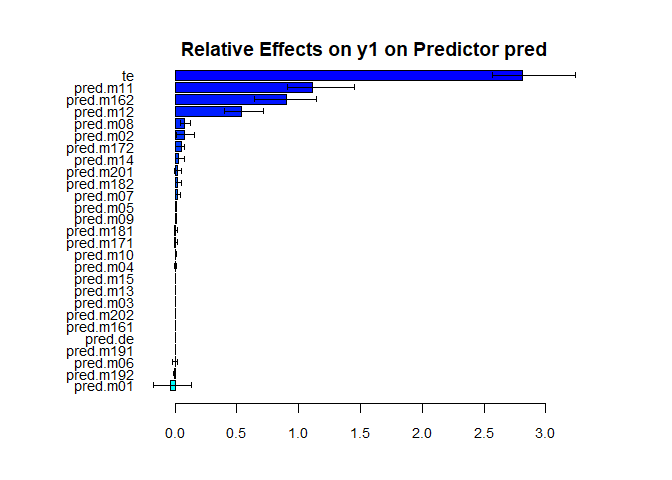mma.big also accepts the organized dataset from data.org.big as the first argument.

mma.e1.2<-mma.big(data=data.e1.2,alpha1=0.05,alpha2=0.05,n2=3)
#>  1
#>  2
#>  3
summary(mma.e1.2,RE=TRUE)
#> The relative effects:
#>
#> For the response variable,y,
#>        pred.de pred.m161 pred.m162 pred.m11 pred.m12 pred.te
#> est      0.007     0.000     0.361    0.442    0.190       1
#> mean     0.058     0.004     0.307    0.458    0.173       1
#> sd       0.100     0.005     0.051    0.112    0.035       0
#> upbd_q   0.165     0.009     0.355    0.551    0.198       1
#> lwbd_q   0.000     0.000     0.259    0.342    0.137       1
#> upbd     0.203     0.009     0.461    0.662    0.258       1
#> lwbd    -0.189    -0.009     0.262    0.222    0.122       1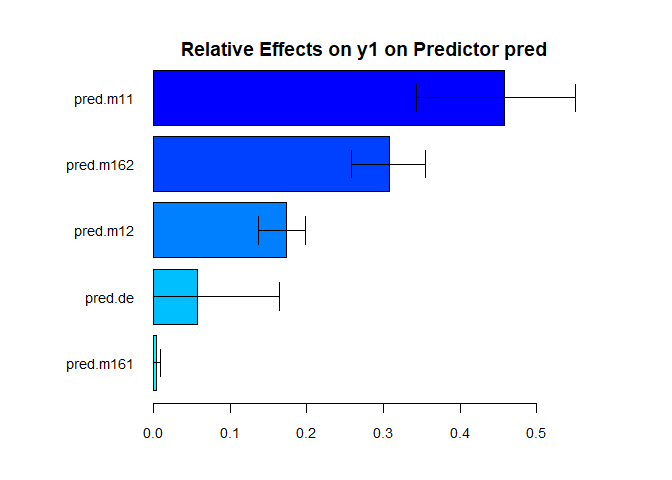### Plots of the fitted mma object from boot.med

plot.mma.big() plots the marginal effect of the selected variable on the outcome, and the marginal effect of the predictor on the selected variable.

#plot(mma.e1.2,vari="m16")
plot(mma.e1.2,vari="m11")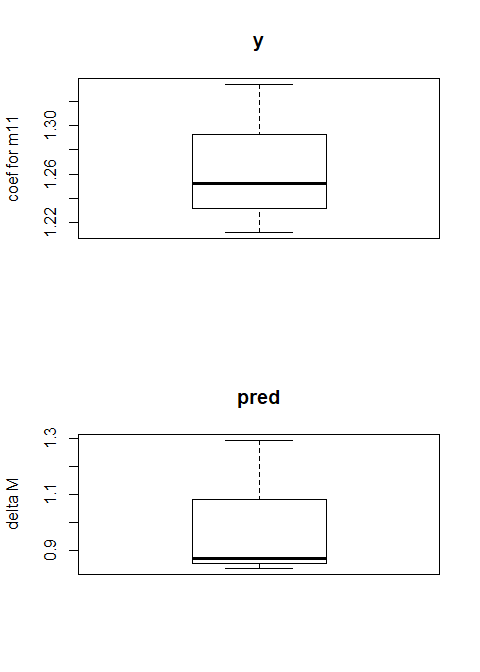In the above figure, the upper panel shows the coefficient of “m11” when it is used to estimate y in an elasticnet regression with y being the outcome in bootstrap samples. We see that the coefficient is significantly positive. The lower panel shows when the exposure variable increases by 1 unit, the average change in “m11”. Again we see that the change in “m11” is positive. Therefore, there is a significant indirect effect (positive) through “m11”. As is shown by summary results, all confidence intervals for the indirect effect of “m11” are to the right of 0.

### Survival outcome

lambda=1/500
survt=-log(runif(n))/lambda/exp(lu)
st=round(runif(n,1,500),0)
time=ifelse(st+survt>600,600,st+survt)-st
cen=ifelse(st+survt>600,0,1)
y=Surv(time,cen)

mma.e3<-mma.big(x=m,y=data.frame(y),mediator=1:ncol(m),pred=data.frame(pred),alpha=1,alpha1=0.05,
alpha2=0.05,n2=3)  #use only the test results.
#>  1
#>  2
#>  3
mma.e3.2<-mma.big(data=data.e3.2,alpha1=0.05,alpha2=0.05,n2=3)  #use only the test results.
#>  1
#>  2
#>  3
summary(mma.e3)
#>
#> The mediaiton effects:
#>
#> For the response variable,y,
#>        pred.de pred.m161 pred.m162 pred.m171 pred.m172 pred.m181 pred.m182
#> est      0.000         0     0.659     0.000     0.000     0.008     0.022
#> mean     0.053         0     0.753     0.003     0.004     0.004     0.012
#> sd       0.092         0     0.431     0.008     0.007     0.007     0.021
#> upbd_q   0.151         0     1.156     0.011     0.011     0.012     0.035
#> lwbd_q   0.000         0     0.337    -0.002     0.000     0.000     0.000
#> upbd     0.180         0     1.504     0.015     0.013     0.022     0.064
#> lwbd    -0.180         0    -0.187    -0.015    -0.013    -0.005    -0.020
#> upbd_b   0.000         0     0.770     0.000     0.011     0.000     0.037
#> lwbd_b   0.000         0     0.314    -0.003     0.000     0.000     0.000
#>        pred.m191 pred.m192 pred.m201 pred.m202 pred.m01 pred.m02 pred.m03
#> est        0.000         0     0.041     0.000    0.052   -0.011        0
#> mean       0.033         0     0.005     0.007    0.006    0.063        0
#> sd         0.050         0     0.010     0.011    0.014    0.117        0
#> upbd_q     0.086         0     0.016     0.019    0.020    0.187        0
#> lwbd_q     0.000         0     0.000     0.000   -0.004   -0.027        0
#> upbd       0.097         0     0.060     0.022    0.079    0.219        0
#> lwbd      -0.097         0     0.023    -0.022    0.026   -0.241        0
#> upbd_b     0.009         0     0.016     0.020    0.000    0.023        0
#> lwbd_b     0.000         0     0.000     0.000   -0.004   -0.029        0
#>        pred.m04 pred.m05 pred.m06 pred.m07 pred.m08 pred.m09 pred.m10
#> est       0.011    0.000    0.000    0.000    0.000   -0.004    0.000
#> mean     -0.002    0.005    0.000    0.004    0.003    0.003    0.004
#> sd        0.010    0.007    0.006    0.007    0.015    0.004    0.026
#> upbd_q    0.007    0.011    0.006    0.011    0.018    0.008    0.032
#> lwbd_q   -0.013   -0.002   -0.005    0.000   -0.009    0.000   -0.014
#> upbd      0.031    0.014    0.012    0.013    0.029    0.005    0.051
#> lwbd     -0.009   -0.014   -0.012   -0.013   -0.029   -0.013   -0.051
#> upbd_b    0.007    0.011    0.000    0.012    0.019    0.008   -0.006
#> lwbd_b   -0.001    0.008   -0.005    0.000    0.000    0.000   -0.015
#>        pred.m11 pred.m12 pred.m13 pred.m14 pred.m15 pred.te
#> est       0.950    0.552    0.026    0.131    0.029   2.466
#> mean      0.896    0.638    0.000    0.072    0.121   2.688
#> sd        0.475    0.214    0.000    0.062    0.108   1.452
#> upbd_q    1.345    0.866    0.000    0.111    0.205   4.111
#> lwbd_q    0.443    0.491    0.000    0.005    0.008   1.357
#> upbd      1.880    0.971    0.026    0.253    0.241   5.313
#> lwbd      0.019    0.133    0.026    0.008   -0.182  -0.380
#> upbd_b    0.901    0.543    0.000    0.112    0.208   2.580
#> lwbd_b    0.419    0.489    0.000    0.000    0.000   1.292
summary(mma.e3.2,RE=TRUE,quant=FALSE)
#> The relative effects:
#>
#> For the response variable,y,
#>        pred.de pred.m161 pred.m162 pred.m171 pred.m172 pred.m11 pred.m12
#> est          0     0.000     0.361     0.000     0.018    0.349    0.272
#> mean         0     0.006     0.377     0.013     0.043    0.341    0.221
#> sd           0     0.018     0.020     0.014     0.007    0.120    0.105
#> upbd_q       0     0.025     0.394     0.026     0.051    0.432    0.329
#> lwbd_q       0    -0.008     0.356     0.001     0.038    0.215    0.134
#> upbd         0     0.035     0.400     0.027     0.032    0.583    0.478
#> lwbd         0    -0.035     0.321    -0.027     0.004    0.114    0.067
#>        pred.te
#> est          1
#> mean         1
#> sd           0
#> upbd_q       1
#> lwbd_q       1
#> upbd         1
#> lwbd         1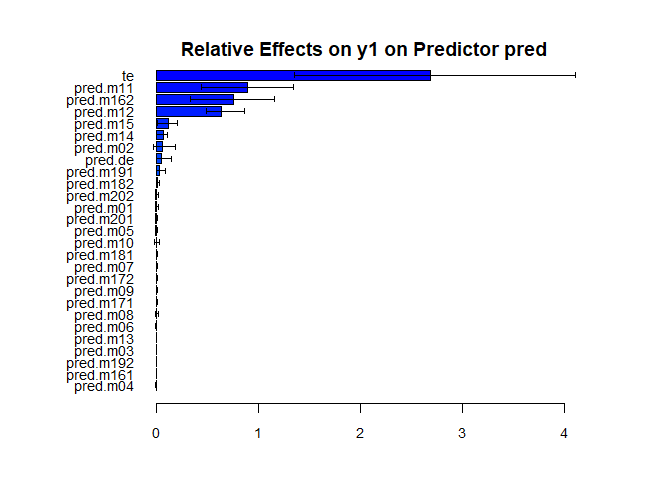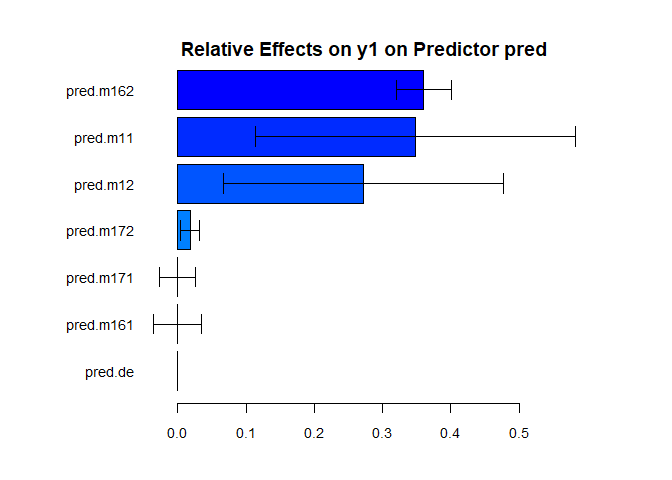We can also plot the selected variables on the survival outcome.

plot(mma.e3.2,vari="m16")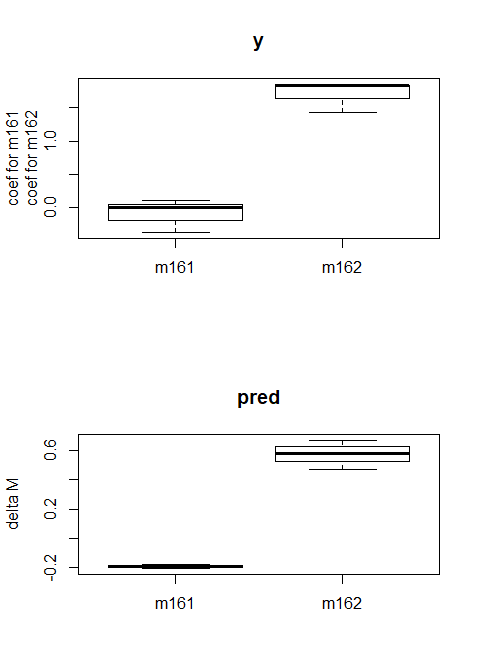plot(mma.e3.2,vari="m11")